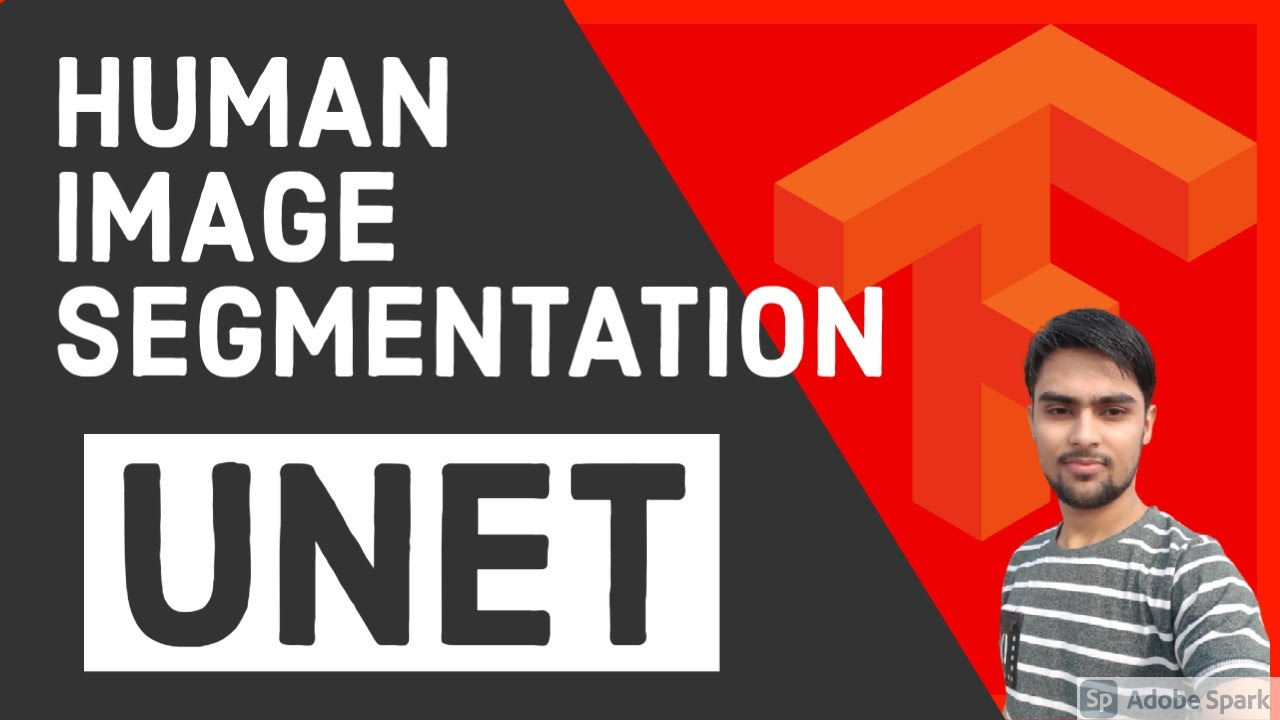# UNet for Person Segmentation || UNet Segmentation using TensorFlow Keras || Deep LearningIn this video, we are going to use the famous UNet architecture for segmenting person from an image. For the person segmentation, we are going to use the person segmentation dataset.

In this video, we are going to use the famous UNet architecture for segmenting person from an image. For the person segmentation, we are going to use the person segmentation dataset.

The U-Net is built for Biomedical Image Segmentation. It is the base model for any segmentation task. It follows an encoder-decoder approach. It used skip connection to get the local information during downsampling path and use it during the upsampling path.

U-Net: https://arxiv.org/abs/1505.04597 ResU-Net: https://arxiv.org/pdf/1711.10684 DoubleU-Net: https://arxiv.org/abs/2006.04868

## Pytorch vs Tensorflow vs Keras | Deep Learning Tutorial (Tensorflow, Keras & Python)

We will go over what is the difference between pytorch, tensorflow and keras in this video. Pytorch and Tensorflow are two most popular deep learning frameworks. Pytorch is by facebook and Tensorflow is by Google. Keras is not a full fledge deep learning framework, it is just a wrapper around Tensorflow that provides some convenient APIs.

## Why Deep Learning is Becoming so Popular? | Deep Learning Tutorial (TensorFlow 2.0, Keras & Python)

This video explains four reasons why deep learning has become so popular in past few years. In this deep learning tutorial python, I will cover following things in this video: Introduction; Data growth; Hardware advancements; Python and opensource ecosystem; Cloud and AI boom

## Keras with TensorFlow Course - Python Deep Learning and Neural Networks

Keras with TensorFlow Course - Python Deep Learning and Neural Networks for Beginners Tutorial: How to use Keras, a neural network API written in Python and integrated with TensorFlow. We will learn how to prepare and process data for artificial neural networks, build and train artificial neural networks from scratch, build and train convolutional neural networks (CNNs), implement fine-tuning and transfer learning, and more!

## top 30 Python Tips and Tricks for Beginners

In this post, we'll learn top 30 Python Tips and Tricks for Beginners

## TensorFlow And Keras Tutorial | Deep Learning With TensorFlow & Keras | Deep Learning

This video on TensorFlow and Keras tutorial will help you understand Deep Learning frameworks, what is TensorFlow, TensorFlow features and applications, how TensorFlow works, TensorFlow 1.0 vs TensorFlow 2.0, TensorFlow architecture with a demo. Then we will move into understanding what is Keras, models offered in Keras, what are neural networks and they work.